In this tutorial, we'll discuss the best libraries for Exploratory Data Analysis in Python.

We will cover these EDA libraries:

Library GitHub Stars Contributors Used by
pandas-profiling 9700 79 9200
D-Tale 3700 22 501
Sweetviz 2200 5 n/a
DataPrep 1400 33 n/a
AutoViz 968 13 265
dabl 684 23 n/a
klib 331 8 n/a

## 1. Overview

Exploratory Data Analysis is a crucial step in the Data Science process.

It not only improves quality and consistency of the data, but it also reveals hidden trends and insights.

``````import pandas as pd
file = 'https://raw.githubusercontent.com/softhints/Pandas-Exercises-Projects/main/data/food_recipes.csv'

``````

• opening the notebook from:
• watching video:

## 2. sweetviz - quick summary

SweetViz generates beautiful and detailed reports with visualizations.
The report is available as HTML output.

### Resources

sweetviz

In-depth EDA (target analysis, comparison, feature analysis, correlation) in two lines of code!

• `pip install sweetviz`

github - sweetviz

### Features

• Target analysis
• Visualize and compare
• Mixed-type associations
• Type inference
• Summary information

### Code

``````import sweetviz as sv

my_report = sv.analyze(df)
my_report.show_html()
``````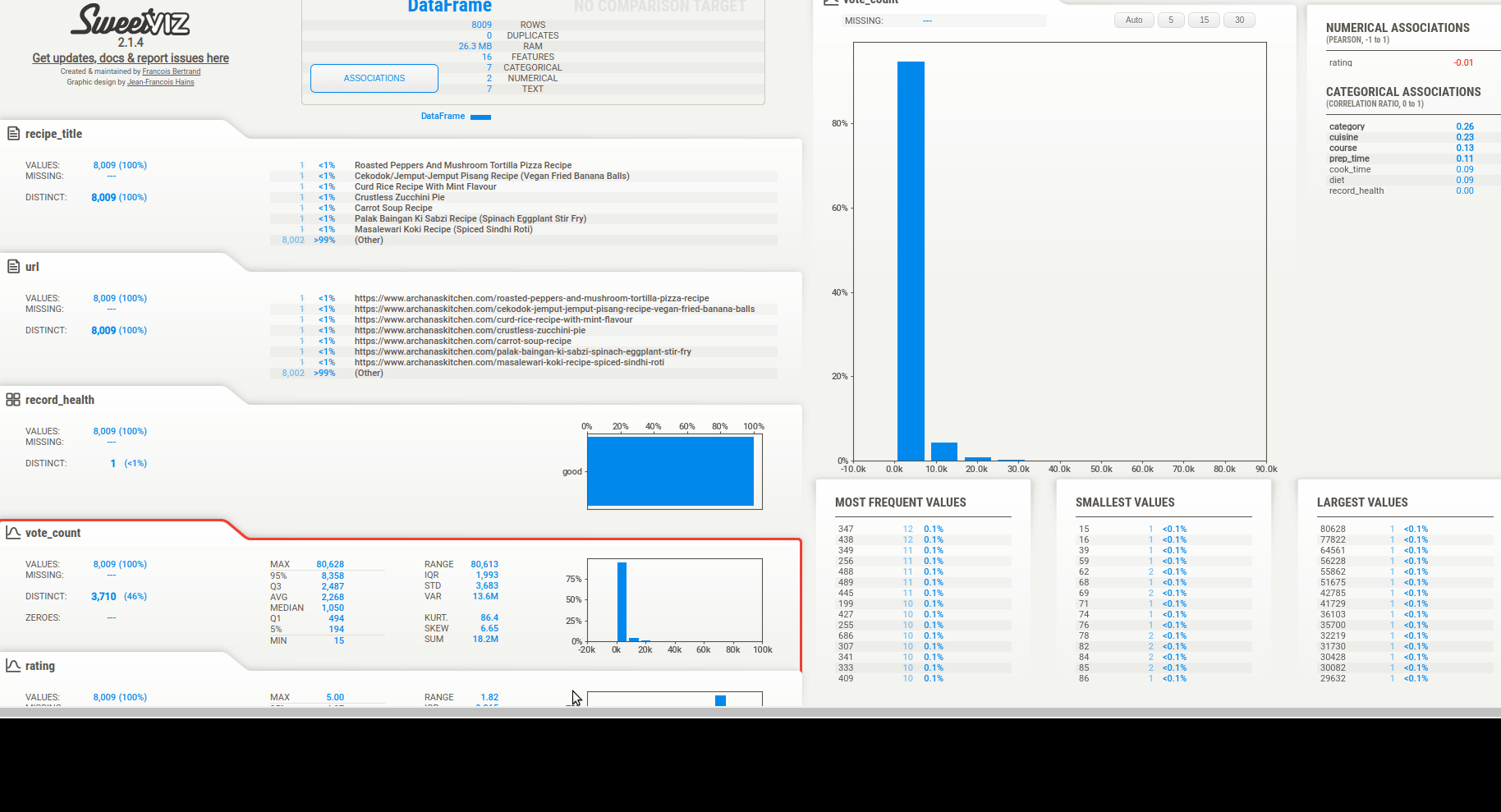We can get quick summary of data to find:

• trends
• missing values
• correlations
• categorical data

We can see a summary of the data types.

Then we can see the associations. There are 3 types of info depending on the column type:

• text
• numeric
• categorical

## 3. autoviz - visualization

As the name suggests it will automatically visualize datasets.
One difference to the first one is that:

• it will take file as an input
• so you need to provide file path and separator
• It's a bit slower than previous one

Reports are generated by using Bokeh as Jupyter output.

### Resources

autoviz

Automatically Visualize any dataset, any size with a single line of code. Now you can save these interactive charts as HTML files automatically with the "html" setting.

• `pip install autoviz`

github - autoviz

### Features

• Visualize and compare
• Summary information
• Outliers and missing values
• Data cleaning improvement suggestions

### Code

``````from autoviz.AutoViz_Class import AutoViz_Class

AV = AutoViz_Class()

#EDA using Autoviz
dft = AV.AutoViz( file, sep=",")
``````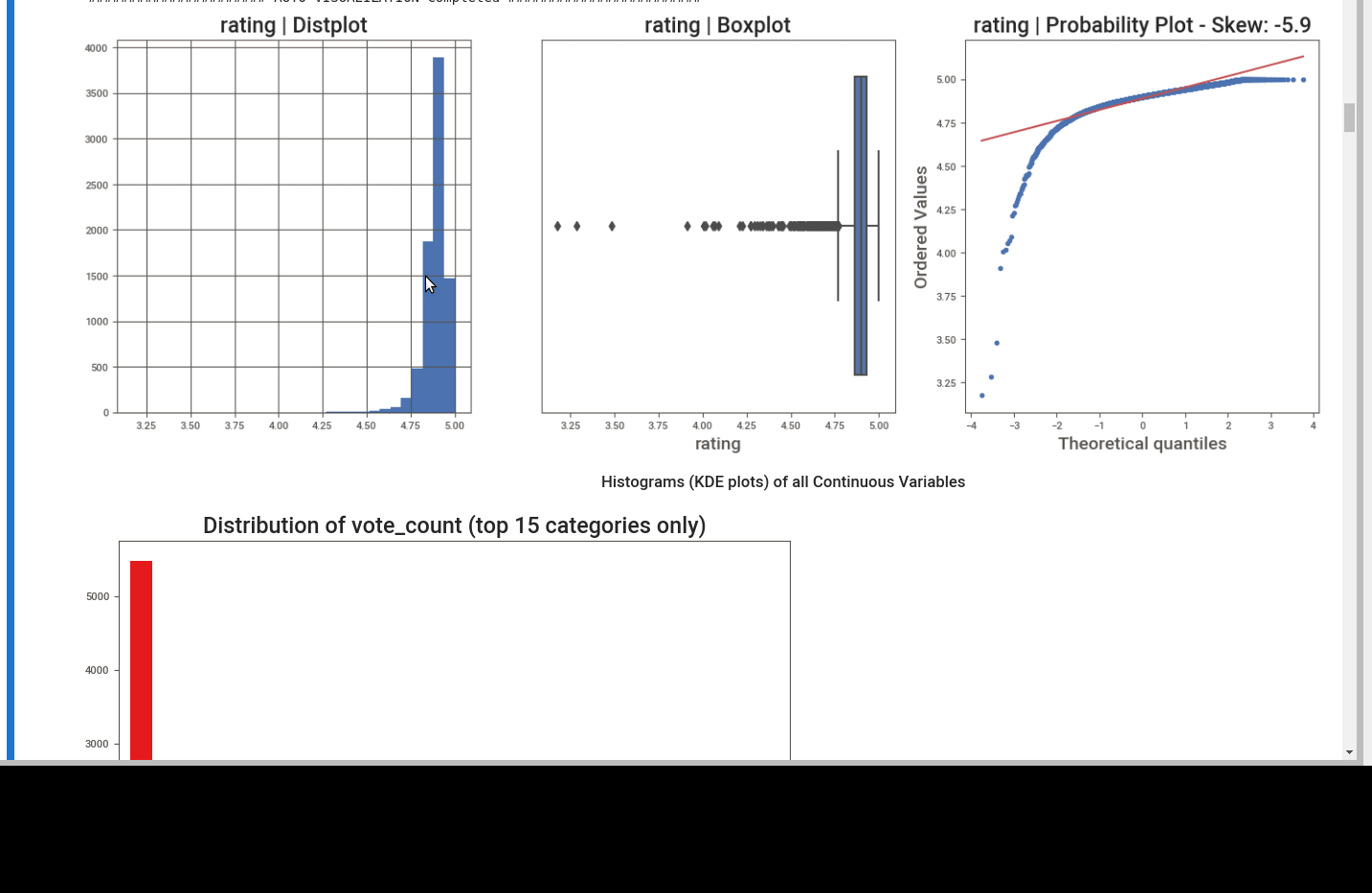First we can see the summary. Number of unique values, missing ones and dtypes.

We know that: prep_time and cook_time are recognized as string columns. But they can be converted to numerical - by replacing ' M'.

This library offers - Data cleaning improvement suggestions. This is very useful for beginners.

We can see different plots depending on the dtype like:

• distribution plot
• box plot
• heatmaps
• bar plots for continuous data
• and finally word clouds for text data.

## 4. pandas-profiling - reports

pandas-profiling is the most popular and most used library according to Github. It has a big list of features and it's very fast.

There are 7 tabs in the final report.

### Resources

pandas-profiling

pandas-profiling generates profile reports from a pandas DataFrame. pandas-profiling extends pandas DataFrame with df.profile_report(), which automatically generates a standardized univariate and multivariate report for data understanding.

• `pip install pandas-profiling`

github - profiling

### Features

• Type inference: detect the types of columns in a DataFrame
• Essentials: type, unique values, indication of missing values
• Quantile statistics: minimum value, Q1, median, Q3, maximum, range, interquartile range
• Descriptive statistics: mean, mode, standard deviation, sum, median absolute deviation, coefficient of variation, kurtosis, skewness
• Most frequent and extreme values
• Histograms: categorical and numerical
• Correlations: high correlation warnings, based on different correlation metrics (Spearman, Pearson, Kendall, Cramér’s V, Phik)
• Missing values: counts, matrix, heatmap and dendrograms
• Duplicate rows: list of the most common duplicated rows
• Text analysis: most common categories (uppercase, lowercase, separator), scripts (Latin, Cyrillic) and blocks (ASCII, Cyrilic)
• File and Image analysis:

### Code

``````from pandas_profiling import ProfileReport

profile = ProfileReport(df, explorative=True)
profile
``````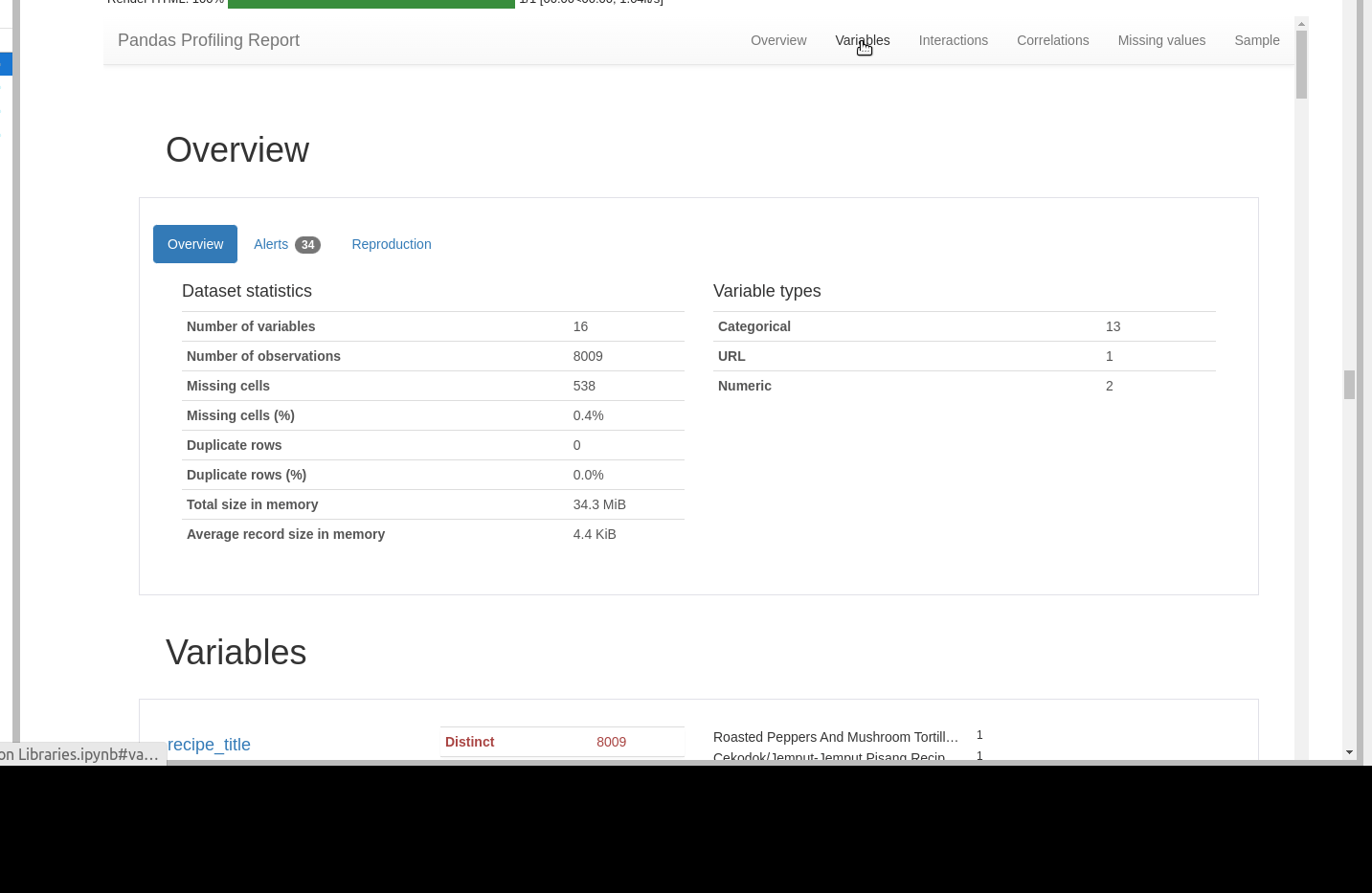#### Overview

In first Tab we can see

• overview of data.
• info for the analysis

#### Variables

• depending on the column type we see different information
• we can get more details by toggle
• URL analysis
• pretty useful feature
• netlocs
• schema
• text data
• min and max length
• most common words
• lower case data
• line breaks etc
• language detection
• most frequent char per script
• numeric
• stats like: 0-s, negative values, mean, max etc
• histogram
• common values
extreme values / possible outliers

#### Interactions

We can find relation between different numeric columns

#### Correlations

There are several methods to get Correlations. We can get a description for each method by clicking on toggle.

#### Missing values

Missing values can be found in several different ways:

• count of null values
• matrix
• heatmap
• dendrogram

There is information below each graph.

#### Sample

In final tab we can see samples from the start and the end of this dataframe

## 5. dataprep - simple EDA

The easiest way to prepare data in Python.

Some functionalities of DataPrep are inspired by Pandas Profiling.

It generates a beautiful profile report from a DataFrame with the create_report function. Works with Pandas and Dask.

### Resources

dataprep

DataPrep lets you prepare your data using a single library with a few lines of code.

• `pip install -U dataprep`

github - dataprep

### Features

• Collect data from common data sources (through dataprep.connector)
• Do your exploratory data analysis (through dataprep.eda)
• Clean and standardize data (through dataprep.clean)

### Code

``````from dataprep.datasets import load_dataset
from dataprep.eda import create_report

create_report(df).show_browser()
``````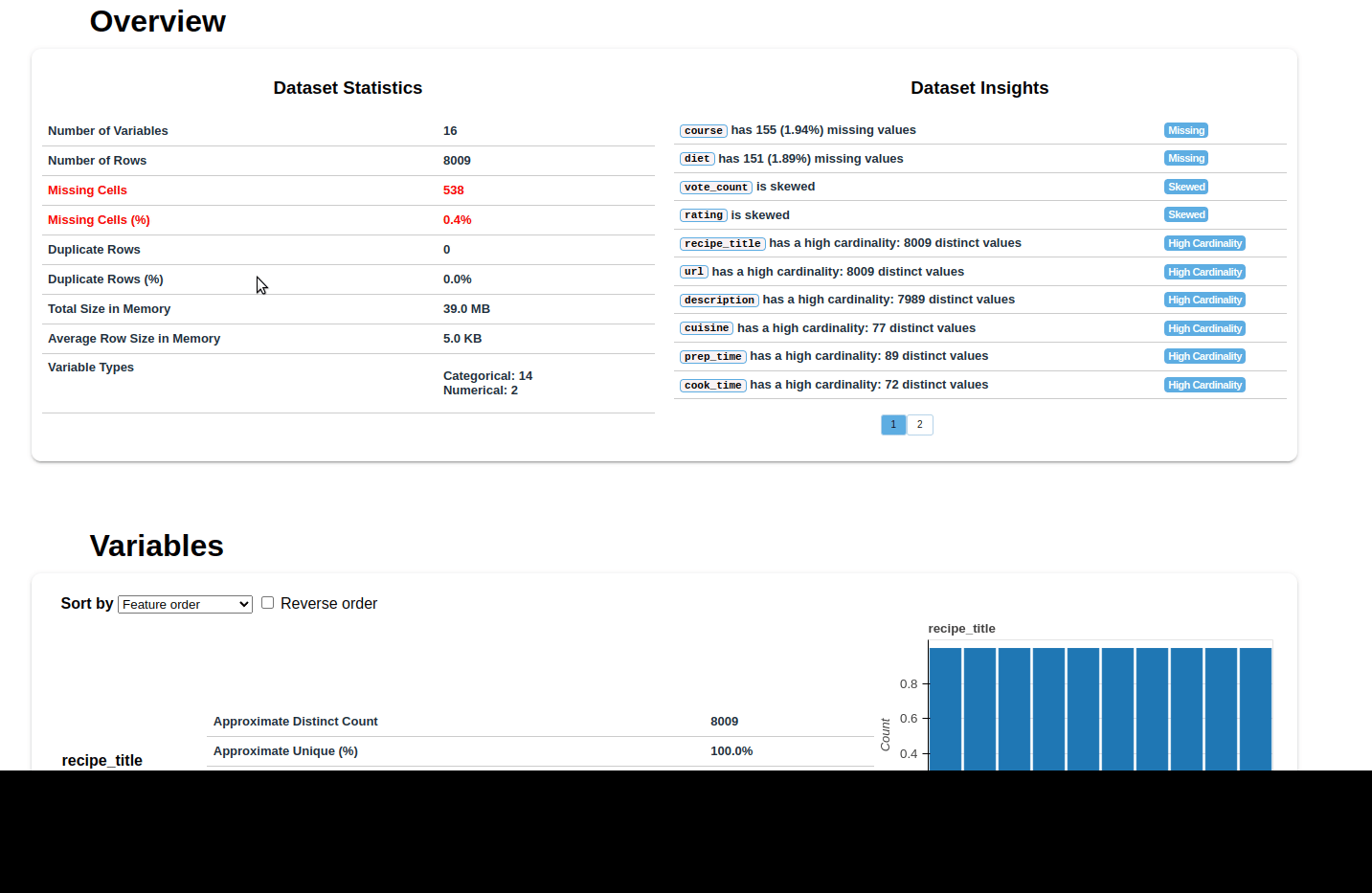• it's very fast due to highly optimized Dask-based computing module
• supports big data
• 140+ functions designed for cleaning and validating data
• clean_country
• validate_country
• plot_correlation
• plot_missing

To list dataprep functions we can use next code snippets:

``````from dataprep.eda import __all__
print(__all__)
``````

which results into:

``````['plot_correlation', 'compute_correlation', 'render_correlation', 'compute_missing', 'render_missing', 'plot_missing', 'plot', 'compute', 'render', 'DType', 'Categorical', 'Nominal', 'Ordinal', 'Numerical', 'Continuous', 'Discrete', 'DateTime', 'Text', 'create_report', 'create_db_report', 'create_diff_report', 'plot_diff', 'compute_diff', 'render_diff']
``````

and

``````from dataprep.clean import __all__
print(__all__)
``````

To get:

``````['clean_lat_long', 'validate_lat_long', 'clean_email', 'validate_email', 'clean_country', 'validate_country', 'clean_url', 'validate_url', 'clean_phone', 'validate_phone', 'clean_json', 'validate_json', 'clean_ip', 'validate_ip', 'clean_headers', 'clean_address', 'validate_address', 'clean_date', 'validate_date',
``````

## 6. dabl - single column

Dabl focuses less on statistical measures of individual columns, and more on providing a quick overview via visualizations, as well as convenient preprocessing.

It's actively developed and not recommended for production.

The goal of dabl is to provide handy tool for beginners which build machine learning modules.

### Resources

dabl

Data Analysis Baseline Library.

• `pip install dabl`

dabl - github

### Features

• Analyze single columns
• Grouped univariate histograms
• Scatter plot for categories
• Determine a good grid shape for subplots
• Create a mosaic plot from a dataframe
• Plots for categorical features in classification
• Visualize coefficients of a linear model

### Code

``````import dabl

dabl.plot(df, target_col="rating")
``````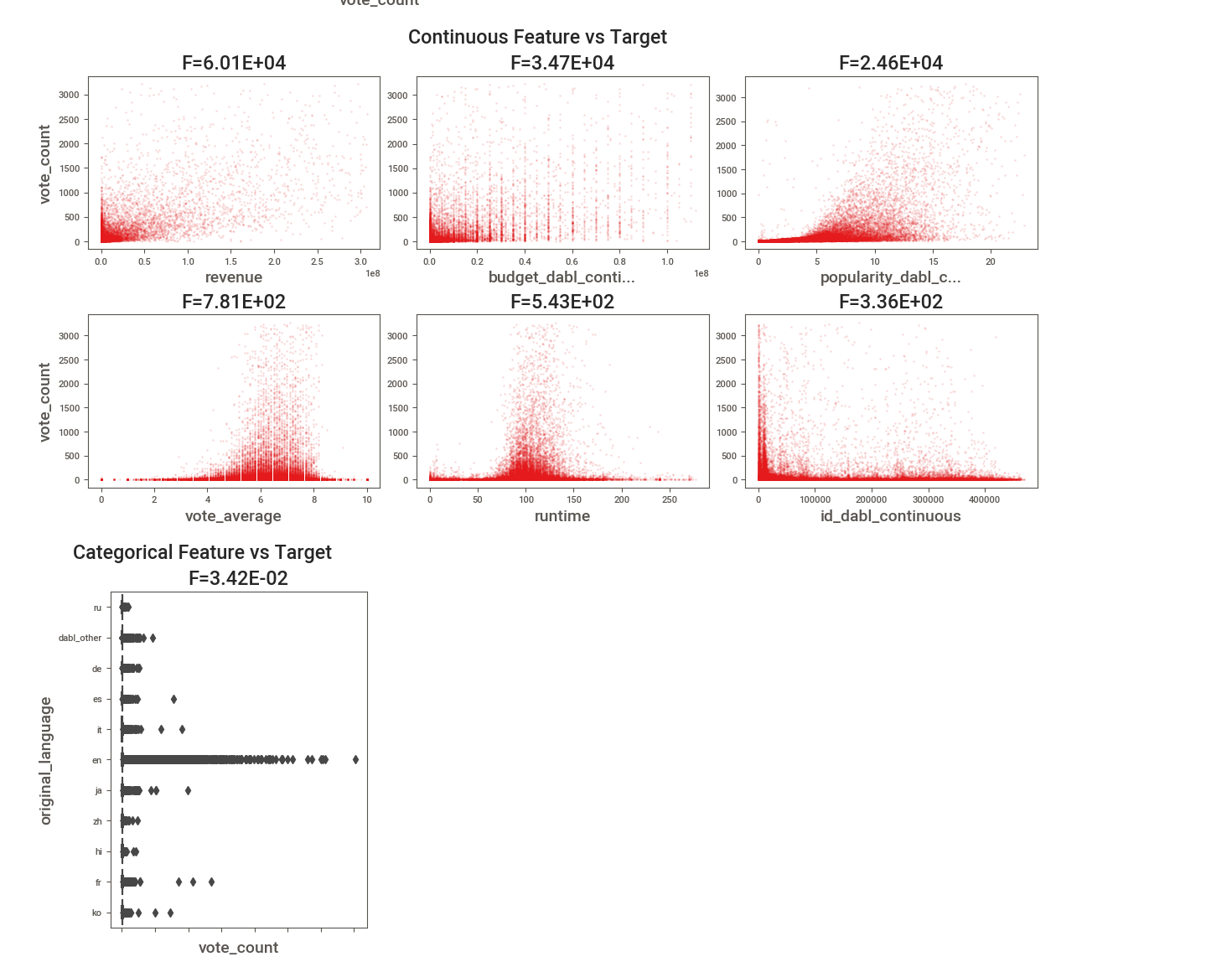## 7. dtale - interactive

D-Tale combines Flask back-end and a React front-end to bring an easy way to view & analyze Pandas data structures.

It's interactive and works with JupyterNotebook and JupyterLab.

Currently this tool supports such Pandas objects as DataFrame, Series, MultiIndex.

dtale

Data Analysis Baseline Library.

• `pip install dtale`

dtale - github

### Features

• Summarize Data
• Duplicates detection
• Missing Analysis
• Outlier Detection
• Custom Filter
• Network Viewer
• Correlations
• Predictive Power Score
• Heat Map
• Load Data & Sample Datasets

### Code

``````import dtale
import pandas as pd

dtale.show(df)
``````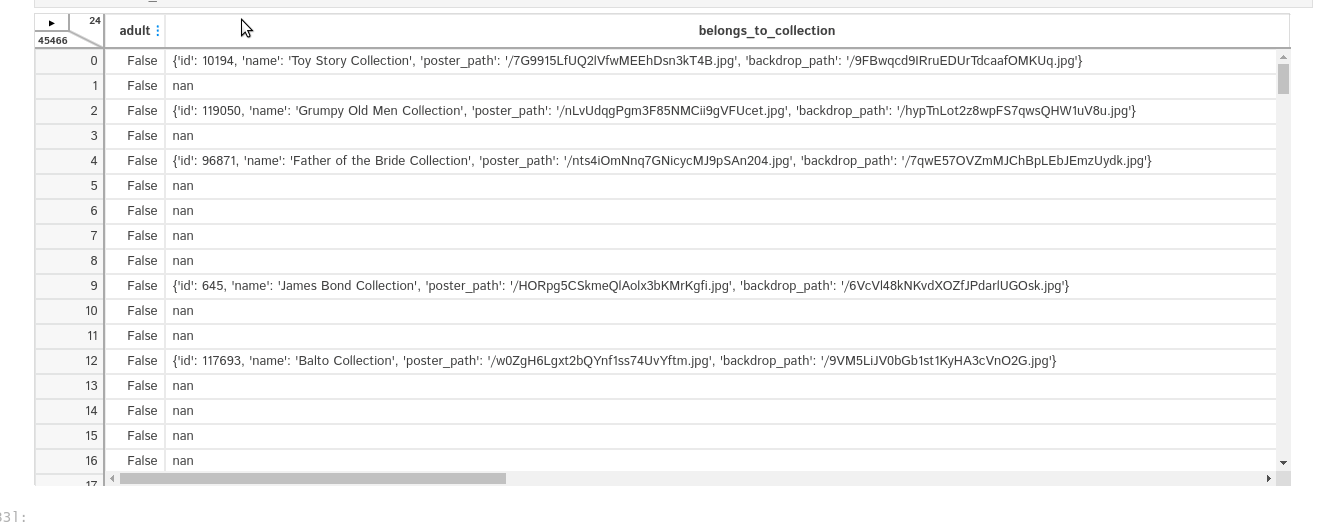## 8. klib - rich features

klib is a library for importing, cleaning, analyzing and preprocessing data. Functions are divided in two areas:

• describe
• clean

klib

Customized data preprocessing functions for frequent tasks..

• `pip install klib`

klib - github

### Features

klib.describe - functions for visualizing datasets

• `klib.cat_plot(df)` - returns a visualization of the number and frequency of categorical features
• `klib.corr_mat(df)` - returns a color-encoded correlation matrix
• `klib.corr_plot(df)` - returns a color-encoded heatmap, ideal for correlations
• `klib.dist_plot(df)` - returns a distribution plot for every numeric feature
• `klib.missingval_plot(df)` - returns a figure containing information about missing values

klib.clean - functions for cleaning datasets

• `klib.data_cleaning(df)` - performs data cleaning (drop duplicates & empty rows/cols, adjust dtypes,...)
• `klib.clean_column_names(df)` - cleans and standardizes column names, also called inside data_cleaning()
• `klib.convert_datatypes(df)` - converts existing to more efficient dtypes, also called inside data_cleaning()
• `klib.drop_missing(df)` - drops missing values, also called in data_cleaning()
• `klib.mv_col_handling(df)` - drops features with high ratio of missing values based on informational content
• `klib.pool_duplicate_subsets(df)` - pools subset of cols based on duplicates with min. loss of information

### Code

``````import klib

klib.missingval_plot(df_m)
df_cleaned = klib.data_cleaning(df_m)
klib.corr_plot(df_m)
klib.corr_plot(df_cleaned, target='revenue')
klib.dist_plot(df_m)
klib.corr_mat(df_cleaned)
``````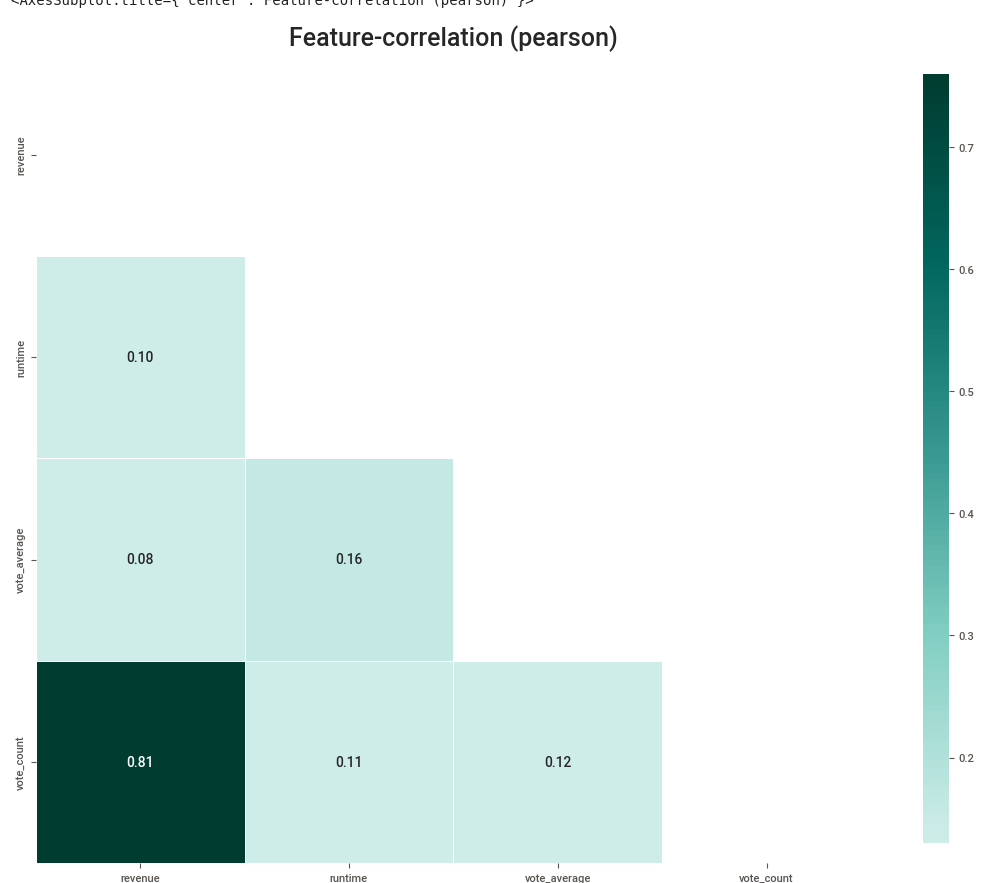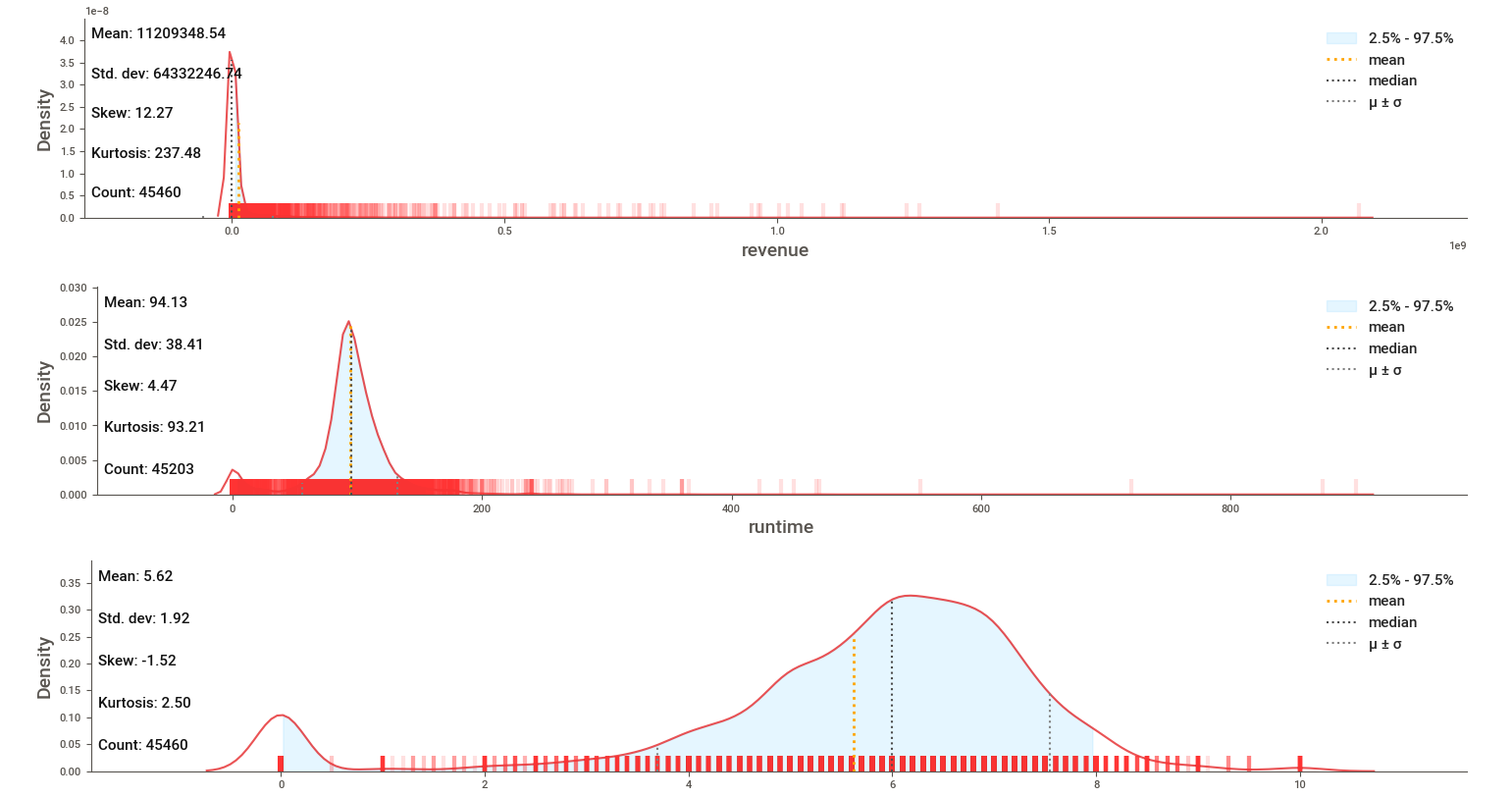## 9. Conclusion

In this article, we explored some of the best libraries of Exploratory Data Analysis and their features in the Python ecosystem.

Using best libraries can help in many aspects of data science process:

• speed up data science projects
• make the optimal decisions
• clean errors and confusion